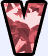# shyvera

Senior Members

36

17 Neutral

• Rank
Quark

## Contact Methods

• Website URL
http://z8.invisionfree.com/DYK/index.php?act=idx

## Profile Information

• Location
London, UK
• Favorite Area of Science
Mathematics
1. Listened to your symphony, it's quite nice. I've been using math in music for a long time and would be interested in some discussions.

2. It's not difficult to plot the graph of [imath]y=||x^2-4x+3|-2|.[/imath] Start by plotting the graph of [imath]y=x^2-4x+3[/imath] and reflecting everything below the x-axis above it. Then drag it down vertically by two units and reflect everything below the x-axis above it again. While graphs themselves do not constitute proofs, they do greatly help you get started. Doing the above, I make it that the graph above intersects the line [imath]y=m[/imath] at exactly 2 points precisely when [imath]m=0[/imath] or [imath]m>2.[/imath] It remains to justify this algebraically – which can be pre
3. I agree. Mathematics can be very useful as a tool for analysing theories of music, but if you want to compose decent music, you still have to understand the rules of harmony, modulation, etc. By the way, I composed a short symphony a few years ago; I call it my Short Symphony in E Minor. It's in three movements, the second of which leads without a break into the finale. I've made a MIDI version of it; see what you think. http://people.delphi...Symphony2_1.mid http://people.delphi...mphony2_2-3.mid
4. A moment ago, I posted something on the application of group theory to music in the thread real life applications of group theory. Here is an elaboration of that post, and another application of maths to music. Circle of fifths I mentioned in my previous post that the theory of intervals is based on the cyclic group of order 12. Here is another way of looking at musical intervals. It's a bit more complicated and less intuitive to the non-mathematical musician as it involves more mathematics – which suits people like me much better. Consider the set of all musical notes distinguished
5.## real life applications of group theory

I know of an application of group theory to music theory. The chromatic scale of Western music consists of 12 notes: C, C#, D, D#, E, F, F#, G. G#. A, A#, B. An interval is the distance from one note to the another – e.g. C–C# is an interval of a semitone, C–D is a whole-tone interval, C–D# is an interval of a minor third, etc. Note that the starting note can be any note, so F–F# is also a semitone interval. The unison interval is the interval from one to itself (e.g. C–C). All intervals that are whole octaves can be identified with the unison interval. Intervals can be “added”, the re
6. A friend of mine suffering from depression is currently on Citalopram treatment. He is a bit uneasy about the drug and is particularly worried that it might make him suicidal. I have looked the drug on Wikipedia (http://en.wikipedia.org/wiki/Citalopram) according to which my friend should be safe as the drug only induces suicidal tendencies in patients under 24 (while my friend is over 50). Does anyone have anything about Citalopram to add to the Wikipedia article? My friend would be happy to know more about the drug.
7. Love waves are dangerous. They are actually earthquake waves! http://z8.invisionfr...p?showtopic=912 They have nothing to do with love, but are named after the English mathematician A.E.H. Love (1863–1940), who developed a mathematical model of these surface waves.
8. I’ve corrected it. By the way, ajb also left out a zero when expanding brackets: (100 + 4)(100 + 4) should be 10000 + 2(400) + 42.
9. By manipulating well-known algebraic formulas, you can devise your own handy arithmetic rules for mental calculation. For example, suppose you want to calculate 91 × 89 mentally. 91 × 89 = (90 + 1)(90 − 1) = 902 − 12 = 8099 Similarly, to do 122 × 118 mentally: 122 × 118 = (120 + 2)(120 − 2) = 14400 − 4 = 14396 You can also do this for division. For example, what is 22491 ÷ 153? Using the trick above, you should be able to get the answer 147 without using a calculator or long division. Now suppose you want to find the prime factors of 359999. If you start by observing t
10.$\frac{\mathrm d}{\mathrm dx}\left[\arcsin\left(\frac{x-a}{x+a}\right)\right]$ $=\ \frac{\mathrm d}{\mathrm dx}\left[\arcsin\left(1-\frac{2a}{x+a}\right)\right]$ $=\ \frac1{\sqrt{1-\left(1-\frac{2a}{x+a}\right)^2}}\cdot\frac{2a}{(x+a)^2}$ $=\ \frac{2a}{(x+a)^2\sqrt{\frac{4a}{x+a}-\frac{4a^2}{(x+a)^2}}}$ $=\ \frac{2a}{(x+a)^2\cdot\frac{\sqrt{4a(x+a)-4a^2}}{x+a}}$ $=\ \frac{2a}{(x+a)\sqrt{4ax}}$ $=\ \frac a{(x+a)\sqrt{ax}}$ $=\ \frac{\sqrt a\sqrt a}{(x+a)\sqrt a\sqrt x}$ $11.## Integrating trig equations Why would you insist on integrating when you could just differentiate? The question doesn’t specifically ask you to integrate; it merely says: Show that [math]\int\mbox{LHS d}x\ =\ \mbox{RHS}.$ So, if you can show that $\frac{\mbox d}{\mbox dx}(\mbox{RHS})\ =\ \mbox{LHS},$ you have answered the question.
12.## Integrating trig equations

Just differentiate the right-hand side.
13. That won’t help. Further hint: $6a^4-2a^3-2a^2b+a^2-6a^2b+2ab+2b^2-b\ =\ \left(a^2-b\right)(\cdots)$
14.## Need someone to point me in the right direction

I would suggesting letting H be the Sylow 11-subgroup (which is certainly characteristic since it’s unique) and K be the centre of H. K is definitely nontrivial (the centre of any p-group where p is a prime is in general nontrivial). Thus if K is a proper subgroup of H, the problem would be solved since the centre of any group is a characteristic subgroup. This leaves the case K = H (i.e. H is Abelian); again this would not be a problem unless H is an elementary Abelian group (which would not have any proper nontrivial characteristic subgroups).
×